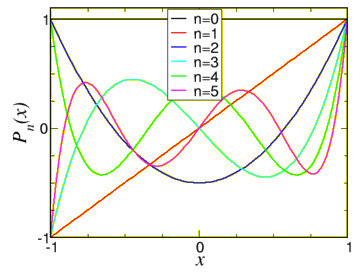$$\newcommand{\id}{\mathrm{id}}$$ $$\newcommand{\Span}{\mathrm{span}}$$ $$\newcommand{\kernel}{\mathrm{null}\,}$$ $$\newcommand{\range}{\mathrm{range}\,}$$ $$\newcommand{\RealPart}{\mathrm{Re}}$$ $$\newcommand{\ImaginaryPart}{\mathrm{Im}}$$ $$\newcommand{\Argument}{\mathrm{Arg}}$$ $$\newcommand{\norm}{\| #1 \|}$$ $$\newcommand{\inner}{\langle #1, #2 \rangle}$$ $$\newcommand{\Span}{\mathrm{span}}$$

# 11.1: Modelling the Eye

$$\newcommand{\vecs}{\overset { \rightharpoonup} {\mathbf{#1}} }$$ $$\newcommand{\vecd}{\overset{-\!-\!\rightharpoonup}{\vphantom{a}\smash {#1}}}$$$$\newcommand{\id}{\mathrm{id}}$$ $$\newcommand{\Span}{\mathrm{span}}$$ $$\newcommand{\kernel}{\mathrm{null}\,}$$ $$\newcommand{\range}{\mathrm{range}\,}$$ $$\newcommand{\RealPart}{\mathrm{Re}}$$ $$\newcommand{\ImaginaryPart}{\mathrm{Im}}$$ $$\newcommand{\Argument}{\mathrm{Arg}}$$ $$\newcommand{\norm}{\| #1 \|}$$ $$\newcommand{\inner}{\langle #1, #2 \rangle}$$ $$\newcommand{\Span}{\mathrm{span}}$$ $$\newcommand{\id}{\mathrm{id}}$$ $$\newcommand{\Span}{\mathrm{span}}$$ $$\newcommand{\kernel}{\mathrm{null}\,}$$ $$\newcommand{\range}{\mathrm{range}\,}$$ $$\newcommand{\RealPart}{\mathrm{Re}}$$ $$\newcommand{\ImaginaryPart}{\mathrm{Im}}$$ $$\newcommand{\Argument}{\mathrm{Arg}}$$ $$\newcommand{\norm}{\| #1 \|}$$ $$\newcommand{\inner}{\langle #1, #2 \rangle}$$ $$\newcommand{\Span}{\mathrm{span}}$$

Let me model the temperature in a simple model of the eye, where the eye is a sphere, and the eyelids are circular. In that case we can put the $$z$$-axis straight through the middle of the eye, and we can assume that the temperature does only depend on $$r,\theta$$ and not on $$\phi$$. We assume that the part of the eye in contact with air is at a temperature of $$20^{\circ}$$ C, and the part in contact with the body is at $$36^{\circ}$$ C.Figure $$\PageIndex{1}$$: The temperature on the outside of a simple model of the eye

If we look for the steady state temperature it is described by Laplace’s equation,

${ \nabla}^{2} u(r,\theta) =0.$

Expressing the Laplacian $${ \nabla}^{2}$$ in spherical coordinates (see chapter 7) we find

$\frac{1}{r^{2}}\dfrac{\partial}{\partial r}{~}\left(r^{2}\dfrac{\partial}{\partial r} u \right) +\frac{1}{r^{2}\sin\theta} \dfrac{\partial}{\partial \theta}{~}\left(\sin\theta\dfrac{\partial}{\partial \theta} u\right) =0.$

Once again we solve the equation by separation of variables,

$u(r,\theta) = R(r) T (\theta).$

After this substitution we realize that

$\frac{[r^{2} R']'}{R} = -\frac{[\sin\theta T']'}{T\sin\theta} = \lambda.$

The equation for $$R$$ will be shown to be easy to solve (later). The one for $$T$$ is of much more interest. Since for 3D problems the angular dependence is more complicated, whereas in 2D the angular functions were just sines and cosines.

The equation for $$T$$ is

$[\sin\theta T']'+\lambda T\sin\theta=0.$

This equation is called Legendre’s equation, or actually it carries that name after changing variables to $$x= \cos\theta$$. Since $$\theta$$ runs from $$0$$ to $$\pi$$, we find $$\sin \theta >0$$, and we have

$\sin\theta = \sqrt{1-x^{2}}.$

After this substitution we are making the change of variables we find the equation ($$y(x)=T(\theta)=T(\arccos x)$$, and we now differentiate w.r.t. $$x$$, $$d/d\theta = - \sqrt{1-x^{2}}d/dx$$)

$\frac{d}{dx}\left[(1-x^{2})\frac{dy}{dx}\right] + \lambda y = 0.$

This equation is easily seen to be self-adjoint. It is not very hard to show that $$x=0$$ is a regular (not singular) point – but the equation is singular at $$x=\pm 1$$. Near $$x=0$$ we can solve it by straightforward substitution of a Taylor series,

$y(x) = \sum_{j=0} a_{j} x^{j}.$

We find the equation

$\sum_{j=0}^{\infty}j(j-1) a_{j} x^{j-2} -\sum_{j=0}^{\infty}j(j-1) a_{j} x^{j} -2\sum_{j=0}^{\infty}j a_{j} x^{j} + \lambda \sum_{j=0}^{\infty} a_{j} x^{j} = 0$

After introducing the new variable $$i=j-2$$, we have

$\sum_{j=0}^{\infty}(i+1)(i+1) a_{i+2} x^{i} -\sum_{j=0}^{\infty}[j(j+1)-\lambda] a_{j} x^{j}=0.$

Collecting the terms of the order $$x^{k}$$, we find the recurrence relation $a_{k+2} = \frac{k(k+1)-\lambda}{(k+1)(k+2)} a_{k}.$

If $$\lambda=n(n+1)$$ this series terminates – actually those are the only acceptable solutions, any one where $$\lambda$$ takes a different value actually diverges at $$x=+1$$ or $$x=-1$$, not acceptable for a physical quantity – it can’t just diverge at the north or south pole ($$x=\cos\theta=\pm 1$$ are the north and south pole of a sphere).

We thus have, for $$n$$ even,

$y_{n} = a_{0}+a_{2}x^{2}+\ldots+a_{n}x^{n}.$

For odd $$n$$ we find odd polynomials,

$y_{n} = a_{1}x+a_{3}x^{3}+\ldots+a_{n}x^{n}.$

One conventionally defines $a_{n} = \frac{(2n)!}{n!^{2} 2^{n}}.$

With this definition we obtain

$\begin{array}{lllllll} P_{0}& =& 1, &~~~&P_{1} & =& x,\\ P_{2}&=& \frac{3}{2}x^{2}-\frac{1}{2},&&P_{3}&=&\dfrac{1}{2}(5x^3-3x),\\ P_4 &=& \frac{1}{8}(35x^{4}-30x^{2}+3) ,&& P_5 &=& \frac{1}{8}(63x^{5}-70x^{3}+15x) . \end{array}$

A graph of these polynomials can be found in figure $$\PageIndex{2}$$.Figure $$\PageIndex{2}$$: The first few Legendre polynomials $$P_n(x)$$.